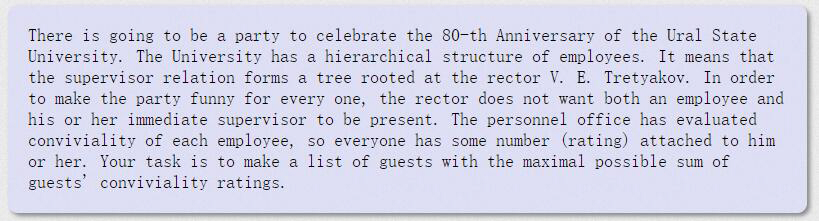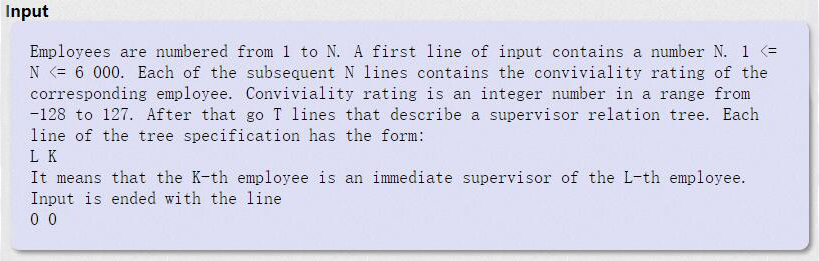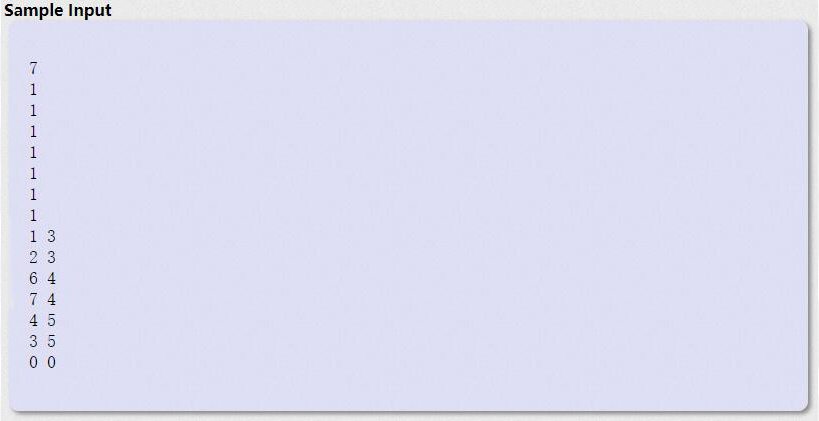# HDU1520 Anniversary party[树形DP]

## A - Anniversary party

HDU - 1520① 上司不去，那么看该下属去的时候的值比较大，还是不去的时候比较大。

dp[now]+=max(dp[next],dp[next])

②上司去，那么下属就不能去。

dp[now]+=dp[next]

#pragma comment(linker, "/STACK:102400000,102400000")
#include<iostream>
#include<cstdio>
#include<cstring>
#include<cmath>
#include<string>
#include<algorithm>
#include<queue>
#include<stack>
#include<set>
#include<map>
#include<vector>
using namespace std;
typedef long long ll;
const int N=6e3+5;
int dp[N];//0不去 1去
int fa[N];
vector<int>vc[N];
int n;
void dfs(int root)
{
for (int i=0 ; i<vc[root].size() ; ++i)
{
int next=vc[root][i];
dfs(next);
dp[root]+=dp[next];//上司去了 所以员工不能去
dp[root]+=max(dp[next],dp[next]);//上司不许,员工可以选择去或不去
}
}
int main()
{
while (~scanf("%d",&n))
{
memset(fa,0,sizeof(fa));
memset(dp,0,sizeof(dp));
for (int i=1 ; i<=n ; ++i)
vc[i].clear();
for (int i=1 ; i<=n ; ++i)
scanf("%d",&dp[i]);
int L,K;
int root=1;
while (~scanf("%d%d",&L,&K) && (L+K))
{
fa[L]=K;
vc[K].push_back(L);
root=K;
}
while (fa[root])
root=fa[root];
dfs(root);
printf("%d\n",max(dp[root],dp[root]));
}
return 0;
}